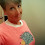## Wednesday, May 25, 2011

### Unit Circle and Wave Functions Project - Part 1

A short while back, I set out to create a GeoGebra project that would help my juniors in Precalculus understand sine and cosine functions. I remembered that over the summer when I was playing with GeoGebra, I had discovered that if you define a point like C = (1, 0), GeoGebra sets the point down statically, but if you first define a slider variable (such as t) and then you define point C using simple trig as C = (cos(t), sin(t)), then GeoGebra creates a point that rotates in a circular path around the origin as you drag to change the value of t along your slider. I remember feeling amazed by this, even though obviously it made total mathematical sense.

So, I sat down in May to create a multi-day project for my Precalc kids, to help the meaning of sine and cosine sink in. It turned out to be pretty darn cool as a learning tool. In the project, the kids had to create several circular points of different radii and rotational speeds (we assumed t meant seconds, and C=(cos(t), sin(t)) thus meant that your point is rotating along a circle at "regular" speed, 1 radian/sec) and directions (clockwise vs. counterclockwise). For each rotating point, they had to create corresponding sine and cosine waves each with a point that traces along the wave as you drag t. This allowed them to verify visually/kinesthetically that that sine is always positive when the circular point is above the x-axis, and cosine is always positive when the circular point is to the right of the y-axis. And then they had to answer a bunch of questions to fully show me that they understand what circular motion is being modeled by
f(x) = 3sin(-0.5x), or g(x) = 0.5cos(x + 3), and WHY and HOW that circular motion impacts the shape of the wave graph.

Anyway. I've got to finish writing about this later. So, consider this the Episode 1 of the unit circle project. You can read about the project specs here. The really cool thing is that my kids submitted their writeup and GGB project files digitally, and so I will get to really show you the results of their understanding.

But, that will come. A bit later. That's Episode 2. Maybe Episode 3 I'll go into then how this feeds into their understanding of solving trig equations. Stay tuned.

1.2.